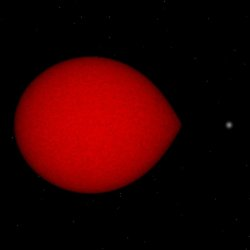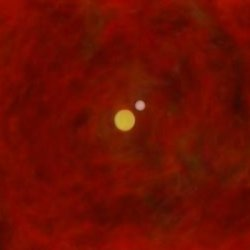# Double white dwarfsDouble white dwarfs are binary stars for which each binary member is a white dwarf. From periodic Doppler shifts in the position of the spectral lines of such a system, one can infer the orbital period. When two sets of spectral lines can be detected, the system is called double lined. At present, ten double-lined white dwarfs are known. Because we have more information for these systems than for single-lined systems, we decided to model these ten double-lined double white dwarfs.

The ten observed systems have masses between 0.3 and 0.7 solar masses (Mo). A striking feature of these systems is that the observed mass ratio is very close to unity, i.e. the two binary members have very similar masses. Although the fact that this is true for all observed systems may be a selection effect, a formation scenario must be able to produce such systems. The orbital periods lie between 90 minutes and a few days, except one system, where Porb=30 days. This means that all orbital separations are between 0.5 and 7 solar radii (Ro), except for the wider system where it is 40Ro. Since white dwarfs of these masses are produced as the cores of red giants with radii measuring hundreds of solar radii, it is clear that a significant orbital shrinkage must have taken place when the last of the two stars made the transition from red giant to white dwarf.The canonical explanation for this orbital shrinkage is that the binary went through a common-envelope (CE) phase. Such a phase can be caused by a giant star that overfills its Roche lobe (the droplet-shaped volume around each binary member, within which gas is bound to only one star) and causes unstable mass transfer resulting in an expanding envelope that soon engulfs the companion star. The drag forces inside the common envelope cause the core of the giant and the secondary star to spiral-in, strongly shrinking the orbit. The orbital energy that is released in this process is used to expand the envelope and unbind it from the system.This last property is often used to compute how much the orbital shrinkage will be: the difference in orbital energy is equated to the binding energy of the giant. The result of such a common envelope is thus a binary consisting of the core of the former giant and the companion star in a much tighter orbit than before (or even a merger of the two stars). We will call this an energy common envelope (E-CE).

When a giant star evolves, its core grows in mass while its radius expands. Thus, there is a relation between the mass of the white dwarf that is formed in a common envelope, which is equal to the core mass of the giant progenitor, and the radius of the progenitor, hence the size of the orbit in which that star filled its Roche lobe. The fact that all observed double white dwarfs have mass ratios close to unity thus suggests that the size of orbit was more or less constant when the first white dwarf was formed, and thus a standard common envelope with energy conservation cannot explain the formation of the first white dwarf.

Nelemans et al. (2000) showed that stable mass transfer does not lead to the desired mass of the first white dwarf either. They suggested to use a common envelope based on angular-momentum balance (AM-CE) for the first envelope-ejection phase rather than energy conservation and showed that the three helium-white-dwarf systems they considered can be explained by one unique efficiency parameter. They used analytic expressions for the core-mass - radius relation of giants and thus had to use an approximation for the binding energy for an E-CE.

We decided to redo the work by Nelemans et al. using a detailed stellar-evolution code. This code not only provides us with more precise values for the stellar radii, it also computes the binding energy of the stellar envelope, which can be used to predict the outcome of an energy common envelope more precisely. Also, the evolution code evolves the stars through helium burning, so that we can consider carbon-oxygen white dwarfs as well as helium white dwarfs. In addition to that, our formation models predict the time that elapses between the formation of the two white dwarfs in the binary, which can be compared to the cooling-age difference, inferred from the observations.

Our work confirms the findings by Nelemans et al. We find that although a few systems might be explained by evolution through stable mass transfer followed by a common envelope, this formation cannot be used for all systems. Where Nelemans et al. used a prescription where mass is lost with the average specific angular momentum of the system, we added two prescriptions where mass is lost with the specific angular momentum of either the donor (i.e. an isotropic wind, d-AM-CE) or of the accretor (isotropic re-emission, a-AM-CE). In these cases, we can demand that the efficiency parameter is close to unity. We find that when the original prescription is used, two successive AM-CEs can explain the observed masses and periods of all ten systems, while an AM-CE followed by an E-CE can explain seven out of ten systems. Our new prescription of a d-AM-CE followed by an a-AM-CE can explain masses and periods of all ten systems, while a d-AM-CE followed by an E-CE can do so for nine out of ten observed double white dwarfs.

Since the orbital period and the mass ratio are the two best-determined observables, and the masses are more uncertain, we demand for a 'good' fit that the period is matched exactly, the mass ratio is matched within the observed range whereas the masses are allowed to be off by up to 0.05Mo. Interestingly, it turns out that the cooling-age difference put a very strict selection criterion on our formation models. When this criterion is applied in addition to that of the period, mass ratio and masses, we find that the combination of d-AM-CE with a-AM-CE gives the best results; out of ten observed systems, it can explain 7 well (age difference within 50% of observed), 2 'marginally' (age difference between 50% and 500% from observed) and one not at all (>500%). The number of systems in each category for d-AM-CE - a-AM-CE is 7/2/1, for the AM-CE - AM-CE scenario it is 6/1/3, for d-AM-CE - E-CE 4/3/3 and for AM-CE - E-CE 4/2/4 (Van der Sluys et al., 2006).

References:
  Nelemans et al., 2000A&A...360.1011N

The images on this page were made by the author, in most cases using Rob Hynes' visualisation program BinSim.